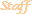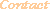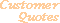Home Older Mathematica Items

Scientific Arts

# Stellar Structure and the Lane-Emden Function: Introduction

Page 1 | Page 2

## Introduction

In Chapter IV of "An Introduction to the Study of Stellar Structure" (Dover, New York, 1958), S. Chandrasekhar discusses the equilibrium configuration of polytropic and isothermal gas spheres. There he derives, in terms of a rescaled temperature,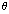, and rescaled radial coordinate,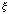, an equation governing. He refers to this as the Lane-Emden equation. It has the form,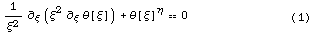Physical constraints at the origin of coordinates require the boundary conditions,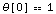(2)Chandrasekhar explicitly solves the Lane-Emden equation for special values of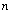: 0, 1, and 5. To do this he invokes a number of clever transformations of Equation 1. For all other cases he indicates that numerical methods must be used.

An important physical parameter associated with the Lane-Emden function is the location of its first positive real zero,: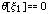. Because the density of the star is related to the temperature via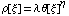, the position of the first zero of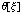corresponds to the surface of the star, and thus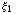gives the radius of the star.

Let's look at the explicit solutions.

### For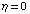:

``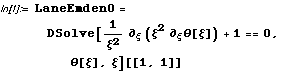``
`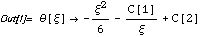`

The boundary conditions of Equation 2 require that the integration constantsand1. Interestingly enough, because of the singularity at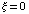, the imposition of the first boundary condition fixes both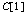and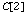: the resulting values also solve the second boundary condition.

Here is the solution.

``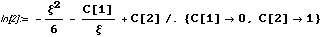``
`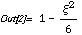`

For this case,.

### For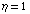:

``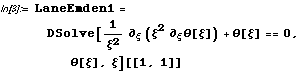``
`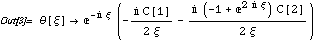`
``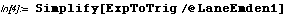``
`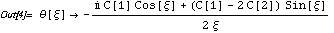`

Here the boundary conditions of Equation 2 require that the integration constants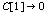and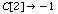. As in thecase, the first boundary condition fixes both constants and those values also satisfy the second boundary condition.

Here is the solution

``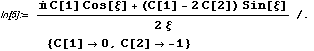``
`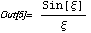`

Here is the second boundary condition .

``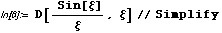``
`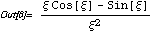`
``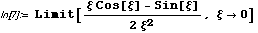``
``

For this case,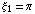.

### For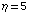:

In this case a number of transformations must be made before the equation yields up its solution. We will not exhibit these here, but you can consult Chandrasekhar's book and use Mathematica to reproduce and verify the manipulations.

For this casehas the form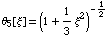and it follows that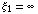.

## Numerical Solution >>

 For further information on our services send email to info@scientificarts.com . Contents of this web site Copyright © 1999-2011, Scientific Arts, LLC.
 s